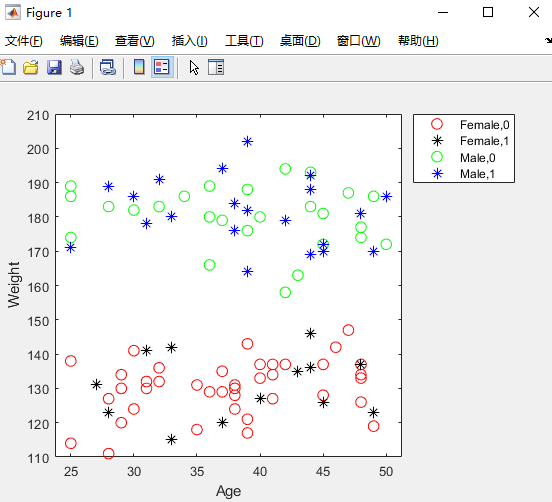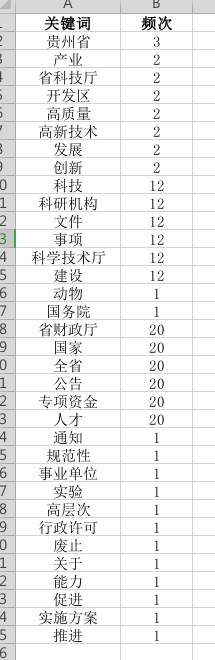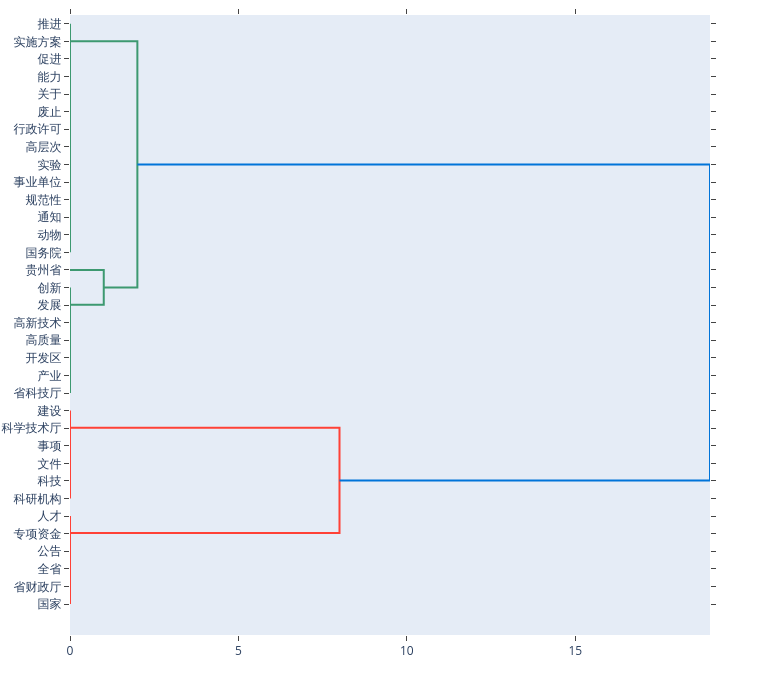• 2022-04-04 17:50:46

# 一、用法

gscatter(x,y,g)
创建 和 的散点图x，y按 分组g。输入x和 y是相同大小的向量。

gscatter(x,y,g,clr,sym,siz)
指定每个组的标记颜色clr、符号 sym和大小siz。

gscatter(x,y,g,clr,sym,siz,doleg)
控制图例是否显示在图表上。 默认创建图例。gscatter

gscatter(x,y,g,clr,sym,siz,doleg,xnam,ynam)
指定用于 x 轴和 y 轴标签的名称。如果您不提供 xnamand ynam，并且 xandy输入是具有名称的变量，则使用变量名称标记轴。gscatter

gscatter(ax,___)
使用轴对象指定的绘图轴ax。指定 ax为第一个输入参数，后跟前面语法中的任何输入参数组合。

h = gscatter(___)
返回与___中的组相对应的图形句柄g。

# 二、clc属性

长名称简称RGB 三元组
黄色的'y'[1 1 0]
品红'm'[1 0 1]
青色'c'[0 1 1]
红色的'r'[1 0 0]
绿色的'g'[0 1 0]
蓝色'b'[0 0 1]
白色的'w'[1 1 1]
黑色的'k'[0 0 0]

# 三、符号属性

价值描述
'o'圆圈
'+'更多迹象
'*'星号
'.'观点
'x'
's'正方形
'd'钻石
'^'向上的三角形
'v'向下三角形
'>'指向右边的三角形
'<'向左三角形
'p'五角星（五角星）
'h'六角星（六角星）
'none'没有标记

如果您没有为所有组指定足够的值，则 根据需要循环指定值

例如：'o+*v'

# 四、案例

load hospital
x = hospital.Age;
y = hospital.Weight;
g = {hospital.Sex,hospital.Smoker};
gscatter(x,y,g,'rkgb','o*',8,'on','Age','Weight')
legend('Location','northeastoutside')MATLAB绘图
更多相关内容
• python绘制聚类树状 import pandas as pd import plotly.figure_factory as ff import chart_studio.plotly as py import chart_studio chart_studio.tools.set_credentials_file(username="用户名", api_key='...

## python绘制聚类树状图

import pandas as pd
import plotly.figure_factory as ff
import chart_studio.plotly as py
import chart_studio
X = data[["总分"]]
fig = ff.create_dendrogram(X, orientation='bottom',labels=list(data["国家"]))
fig['layout'].update({'width':800, 'height':800})
py.iplot(fig, filename='dendrogram_with_labels')注意
需要先注册plotly生成密钥https://plot.ly/settings/api/
之后才可以正常使用，生成的树状图保存到云端文件夹中
欢迎加群：620139909展开全文python
• 何为聚类分析？ 聚类分析指将物理或抽象对象的集合分组为由类似的对象组成的多个类的分析过程。它是一种重要的人类行为。 MATLAB、SPSS等商业软件包中具有聚类分析相关功能，在普通web编程中需要直接在我们程序中...

# 何为聚类分析？

聚类分析指将物理或抽象对象的集合分组为由类似的对象组成的多个类的分析过程。它是一种重要的人类行为。

MATLAB、SPSS等商业软件包中具有聚类分析相关功能，在普通web编程中需要直接在我们程序中使用聚类分析又不想使用商业软件，python的plotly库是一个不错的选择。

聚类分析方法有层次聚类法、K-均值聚类法等，本文为python绘制层次聚类分析图。
层次聚类分析方法的基本思想是：通过某种相似性测度计算节点之间的相似性，并按相似度由高到低排序，逐步重新连接个节点。该方法的优点是可随时停止划分，主要步骤如下：

1. 移除网络中的所有边，得到有n个孤立节点的初始状态；
2. 计算网络中每对节点的相似度；
3. 根据相似度从强到弱连接相应节点对，形成树状图；
4. 根据实际需求横切树状图，获得社区结构。

# 安装plotly及相关依赖

### 0.安装python3环境

安装python3环境网络上资料比较多，请自行安装python3环境

### 1.安装plotly

pip3 install plotly


### 2.安装相关依赖

pip3 install pandas
pip3 install numpy


# 数据集

数据为csv文件（csv格式其实是：逗号分隔值文件格式，可以用记事本或excel打开），示例内容test.csv为：# 完整代码

这里保存的文件名为：myPlotly.py

#!/usr/bin/env python3
# -*- coding: utf-8 -*-

#import chart_studio

import sys
import os
import pandas as pd
#import chart_studio.plotly as py
import plotly.figure_factory as ff
import numpy as np

#数据集，csv格式
csvData="/opt/test.csv"
#输出的html文件路径
outFilePath="out-plotly.html"
#是否在浏览器中打开生成的html文件
autoOpenOnBrowser=False

####function echo() start######
def echo( param,*args ):
if len(args)==0:
print(param)
else:
for var in args:
if var=='':
print(param,end='')
else:
print(param)
####function echo() end#######

############### help start ##########################
def _help():
echo("Usage: %s [Options]" % (sys.argv))
echo("Options:")
echo("    -?,-h,-help,--help \t :this help")
echo("    -csv=csvPath \t :csvPath是数据集csv文件路径")
echo("    -out=outHtmlPath \t :outHtmlPath是输出的html文件路径")
############### help end ##########################
############function: 汉化#######################
def toZhCN(saveFileName):
with open(saveFileName, 'r+') as fo:
if filedata.strip() == '':
return False
filedata = filedata.replace('"Zoom"','"局部放大"')
filedata = filedata.replace('"Pan"','"移动"')
filedata = filedata.replace('Zoom in','放大')
filedata = filedata.replace('Zoom out','缩小')
filedata = filedata.replace('Autoscale','自动缩放')
filedata = filedata.replace('Reset axes','重置')
filedata = filedata.replace('Toggle Spike Lines','切换峰线')
filedata = filedata.replace('Show closest data on hover','在悬停时显示最近的数据')
filedata = filedata.replace('Compare data on hover','比较悬停数据')
filedata = filedata.replace('"https://plotly.com/"','"#"')
filedata = filedata.replace('Produced with Plotly','新窗口打开')
filedata = filedata.replace('plotly-logomark','')
try:
fp=open(saveFileName,"w+",encoding="utf-8")
fp.write(filedata)
finally:
fp.close()
return True
###############func:createPlotly#############
def createPlotly():
try:
X = data[["频次"]]
kwdlist = list(data["关键词"])
kwdsize = len(kwdlist)
fig = ff.create_dendrogram(X, orientation='left',labels=kwdlist)
layoutHeight = round((kwdsize-40)/10)*100+800
if kwdsize<=40:
layoutHeight = 800
fig['layout'].update({'width':800, 'height':layoutHeight})
#py.plot(fig, filename='dendrogram_with_labels')
#输出html
fig.write_html(outFilePath, auto_open=autoOpenOnBrowser)
#fig.show()
if os.path.exists(outFilePath):
toZhCN(outFilePath)
echo("success")
except:
echo("error:" + sys.exc_info())

def main():
global csvData
global outFilePath
if sys.version < '3':
echo("error:请使用Python3")
sys.exit(0)
argvlen=len(sys.argv)
if argvlen==1:
echo("error:参数不能为空，通过 %s -? 查看参数" % sys.argv)
else:
csvDataTmp=""
outFilePathTmp=""
showHelp=False
for ii in range(1,argvlen):
paramData=sys.argv[ii]
if paramData.startswith('-csv='):
csvDataTmp=paramData[5:]
elif paramData.startswith('-out='):
outFilePathTmp=paramData[5:]
elif paramData == '-?' or paramData == '-h' or paramData == '--help' or paramData == '-help' :
showHelp=True
_help()
break
if not showHelp:
if csvDataTmp and os.path.exists(csvDataTmp):
csvData=csvDataTmp
outFilePath=outFilePathTmp
createPlotly()
else:
echo("error:数据集不存在")
if not outFilePathTmp:
echo("error:输出的html文件路径不能为空")

if __name__ == "__main__":
main()


# 使用示例：

python3 myPlotly.py -csv=/opt/test.csv -out=/opt/test.html


生成的结果：展开全文python
• R语言数据可视化_科学统计图表3——ggplot绘制层次聚类图 层次聚类图相信大家都很了解了，但是我们往往只能够画一个十分朴素的层次聚类图，像这样的… 或许我们可以在这张图上稍作修改，但也仅限于在某个高度加条...

## R语言数据可视化_科学统计图表3——ggplot绘制层次聚类图

层次聚类图相信大家都很了解了，但是我们往往只能够画一个十分朴素的层次聚类图，像这样的…或许我们可以在这张图上稍作修改，但也仅限于在某个高度加条红线，这就造成了读图的困难。这个问题之所以困扰我们，是因为并不是所有的绘图函数都如ggplot一样充满艺术性且有非常多的受众（便于我们查找和学习）。就算某个根据ggplot开发的包能够绘制层次聚类图，它也不像ggplot那样能够任由我们天马行空的想象对图自由地进行修改。

所以我（趾高气昂），今天！用ggplot写了个层次聚类，为广大学子带来了福音（掌声）！我并没有打算把它封装成一个包或函数，因为这样做相当于抛弃了ggplot的自由性和艺术性，所以本片文章我会从数据开始，一步步的解释我的代码。

library("factoextra")

data(USArrests)
#这次所用的数据

df <- scale(USArrests)
#标准化一下

result <- dist(df, method = "euclidean")
result_hc <- hclust(d = result, method = "ward.D2")
#获得层次聚类的结果result_hc

fviz_dend(result_hc, cex = 0.6)
#画个层次聚类图看看


然后我们用ggplot重写下
我的绘制思想是利用geom_line画出图中的每一个杠(如下图)，每一个杠由四个点顺序链接所构成，所以代码主要就是在找每个杠对应的四个点，并用group将他们分开。len = dim(USArrests)
#这里定义了数据的数量

#######################################################
################危      从这里开始      危####################
#######################################################

ranks = c(1:(2*len-1))

for (i in c(1:len)) {
ranks[i] = which(result_hc$order==i) } ranks[(len+1):(len+2)] = 0 for (i in 1:(len-1)) { rank1 = 0 rank2 = 0 if(result_hc$merge[i,1]<0)
{
rank1 = ranks[-result_hc$merge[i,1]] }else{ rank1 = ranks[result_hc$merge[i,1]+len]
}

if(result_hc$merge[i,2]<0) { rank2 = ranks[-result_hc$merge[i,2]]
}else{
rank2 = ranks[result_hc$merge[i,2]+len] } ranks[i+len] = (rank1 + rank2)/2 } ranks x = c(1:4*(len-1)) y = c(1:4*(len-1)) heigh = c(1:(2*len -1)) heigh[1:len] = 0 heigh[(len+1):(2*len -1)] = result_hc$height

merge_ = result_hc$merge for (i in 1:(2*(len-1))) { if(result_hc$merge[i]>0)
{
merge_[i] = result_hc$merge[i]+len }else{ merge_[i] = -result_hc$merge[i]
}
}

for (i in 1:(len-1)) {
index = (i-1)*4 + 1
x[index] = ranks[merge_[i,1]]
y[index] = heigh[merge_[i,1]]

x[index+1] = ranks[merge_[i,1]]
y[index+1] =   result_hc$height[i] x[index+2] = ranks[merge_[i,2]] y[index+2] = result_hc$height[i]

x[index+3] = ranks[merge_[i,2]]
y[index+3] =   heigh[merge_[i,2]]

}

#######################################################
################危      到这里结束      危####################
#######################################################
#都不用看！
#复制粘贴就可以

########################################################
data = data.frame(x=x,y=y,group = rep(1:(len-1),each=4))
#这个data是绘制图像所用数据，也是上面那段代码的全部成果

ggplot(data,aes(x=x,y=y,group=group ))+geom_line()
#绘制完成~看下data这里group由低到高分别对应了聚类分析的每次聚类结果，比如说最大的组——group49就对应了图中最高的那个杠…好嘞，看下ggplot能实现什么样的想法吧！有哪里不懂的小伙伴可以私信或评论，另外本人也提供一些科学图表的有偿绘制服务哦~
价格实惠呢亲！展开全文r语言
• 大家好，有朋友在后台问如何用 R 绘制聚类热图，其实绘制聚类图的方法有很多，比如 MeV 软件等等，我们今天看如何用 R 来绘制聚类图。首先我们看原始数据，原始数据有两组，每组 4 个样品，共八个样品，每个样品有 ...前端
• 该资源主要参考我的博客： [python] Kmeans文本聚类算法+PAC降维+Matplotlib显示聚类图像 ...包括输入文档txt，共1000行数据，每行都是...最后调用Matplotlib显示聚类效果。 免费资源，希望对你有所帮助~ By Eastmount
• ] # # #5个簇，1个自由点 # mod = KMeans(n_clusters=5, random_state=1) ##用训练器数据X拟合分类器模型并对训练器数据X进行预测 # y_pre = mod.fit_predict(x) # # #打印聚类的结果 # r1 = pd.Series(mod.labels_)...
• 如截图所示，传递闭包输出分类系数和矩阵后，如何把分析系数作为节点绘制聚类分析树状matlab
• 总结： 在clustermap函数里面其实是使用了heatmap的，但是加上了聚类功能，使得其绘制出来的热图具有横向样本(sample)和纵向基因(gene)的聚类功能，更符合生物信息学分析的要求。 在实际应用过程中，在导入差异基因...
• 在使用Matlab做聚类分析的时候一般这样用： Y = pdist(X); Z = linkage(Y,'single'); dendrogram(Z); 这样调用dendrogram就能够自动生成树状，默认显示30个节点的树状，像下面这样 如果...
• 文章目录引言6.1 K-means聚类6.1.1 SciPy聚类包6.1.2 图像聚类6.1.3 在主成分上可视化图像6.1.4 像素聚类6.2 谱聚类 引言 本章将介绍聚类方法，用图像进行聚类，寻找相似的图像组。聚类可用于识别、划分图像数据集，...
• 在进行机器学习时，我们往往要对数据进行聚类分析，聚类，说白了就是把相似的样品点/数据点进行归类，相似度高的样品点会放在一起，这样一个样本就会被分成几类。而聚类分析也有很多种方法，比如分解法、加入法、...
• import numpy as np import pandas as pd import seaborn as sns from ...g=sns.clustermap(df,center=0,cmap='RdBu',figsize=(10,20)) ax = g.ax_heatmap plt.title=("Embro") plt.savefig("聚类热图.pdf") plt.show()数据挖掘 机器学习
• 而不绘制行标签在不同的坐标轴方向绘制颜色条使用遮罩绘制矩阵中的一部分聚类热图使用不同的相似性指标使用不同的色彩映射并忽略色彩映射限制中的异常值添加彩色标签散点图绘制一个两个变量的简单散点通过其他python 开发语言
• ## 4-2 图像聚类算法

千次阅读 2020-08-28 21:31:32
Means聚类2.1 概念2.2 步骤2.3 例子2.4 K-Means聚类与图像处理2.5 K-Means聚类优缺点优点：缺点3 层次聚类3.1 概念3.2 凝聚层次聚类的流程3.3 层次聚类的优缺点3.4 树状分类判断3.5 K-Means与层次聚类4 密度聚类...
• 1.kmeans肘部和DBSCAN轮廓 import pandas as pd import numpy as np import matplotlib.pyplot as plt from sklearn.cluster import KMeans from sklearn.metrics import silhouette_score # 3.读取drink.txt...机器学习 kmeans
• 一般来说，Origin Pro主要工作就是画图，官方本身是不支持聚类分析的，也就无法绘制聚类分析树状了。据说可以通过origin的App来实现，这一点由于种种原因没有验证过（访问不了官网，无法注册账号，你懂的）。需要...
• 之前使用k均值方法将数据划分到不同的簇中，但当变量个数大于2时，就无法在二维空间中展示数据聚类的过程，因此可以使用二元聚类图先将变量减少成两个主要成分，然后利用组件（诸如轴线和椭圆）来展示数据聚类的结果...r语言
• 我试图研究这个问题，但失败了。我对python很在行，所以请...然后我想把它画在柱状上。我有以下代码：angle = open(output_dir+'/chi_angle.txt', 'r').read().splitlines()array = numpy.array(map(float, ang...
• 34行MATLAB代码实现k均值聚类，包含展示聚类成功后的散点
• centroids = estimator.cluster_centers_ #获取聚类中心 inertia = estimator.inertia_ # 获取聚类准则的总和 mark = ['or', 'ob', 'og', 'ok', '^r', '+r', 'sr', 'dr', ' 效果： 参考文献： 1、python中plot用法...python
• Kmeans聚类算法-手肘法，jupyter notebook 编写，打开可以直接运行，使用iris等5个数据集，机器学习。
• 1、前言聚类分析是机器学习和数据分析中非常常见的分类方法，当我们用到层次聚类（系统聚类）时，最常用的分析方法就是绘制树状，比较常见的统计软件像SPSS、SAS、R等都可以直接绘制树状，比较简单，今天主要...
• SPSS系统聚类输出的树状广受用户喜爱，二阶聚类也可以输出一系列美观的可视化图形用来观察聚类效果，但我们发现Kmeans均值聚类没有提供可视化程度高的图形，那怎么办，我们自己来制作。数据小兵推荐使用3D散点全...
• KMeans聚类绘制聚类后的决策边界 # 导数基础包和函数 from sklearn import datasets import matplotlib.pyplot as plt # 使用skelarn的数据生成工具make_blobs生成聚类仿真数据集； # 对仿真数据进行可视...机器学习 数据挖掘 深度学习 自然语言处理
• Python实现一般使用以下函数，其输出为一个n行4列的矩阵，表示层次聚类后的树状。 tree = scipy.cluster.hierarchy.linkage(arr,'ward') 输出示例如下，前两列代表每一步合并的两个簇，第三列为簇间距离，第...python 数据结构...